NESS ENGINEERING
TECHNICAL DATA
RLC CIRCUIT FORMULAS

The formulas on this page are associated with a series RLC circuit discharge since this is the primary model for most high voltage and pulsed power discharge circuits.

Each of the following waveform plots can be clicked on to open up the full size graph in a separate window.

UnderdampedThe circuit schematic for the underdamped case is shown below. In this specific model, the resistance is an order of magnitude (10X) less than the value required for a critically damped circuit.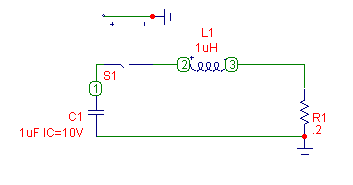The results of the circuit model are shown below. V(1) is the voltage on the 1 mF capacitor as it discharges in an oscillatory mode. V(3) is the voltage on the load resistor, in this case a 0.2 ohm value. The circuit current is graphed in the second, lower plot and reaches its peak value very nearly at t=p/2w0.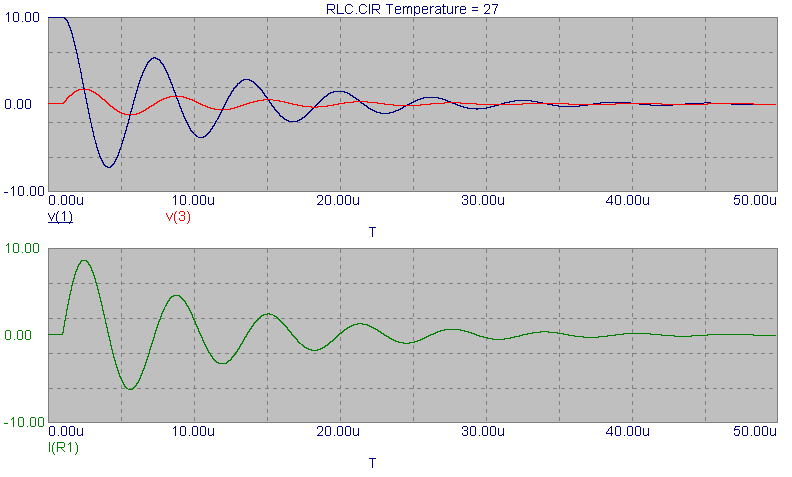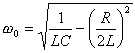Omega L is the circuit inductance (H)  C is the circuit capacitance (F)  R is the circuit resistance (W)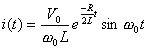Current V0 is the initial voltage on the capacitance (V)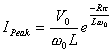Peak Current Same as above

Overdamped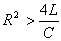The circuit schematic for the overdamped case is shown below. In this specific model, the resistance is an order of magnitude (10X) more than the value required for a critically damped circuit.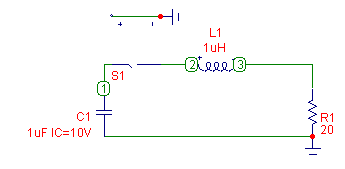The results of the circuit model are shown below. V(1) is the voltage on the 1 mF capacitor as it discharges in a simple RC decay mode. V(3) is the voltage on the load resistor, in this case a 20 ohm value. In this case, once the switch closes and the voltage on the load resistor rises to match the capacitor voltage, both waveforms then essentially overlap and decay at the same rate since the voltage across the inductor is minimal. The circuit current is graphed in the second, lower plot.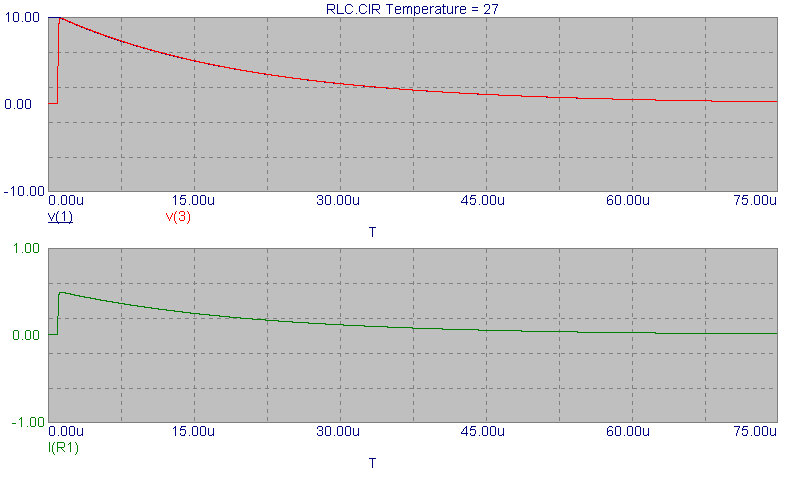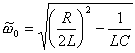Omega L is the circuit inductance (H)  C is the circuit capacitance (F)  R is the circuit resistance (W)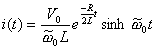Current V0 is the initial voltage on the capacitor (V)

Critically Damped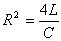The circuit schematic for the critically damped case is shown below. In this specific model, the resistance is exactly equal to the value required for a critically damped circuit.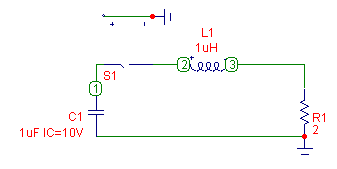The results of the circuit model are shown below. V(1) is the voltage on the 1 mF capacitor as it discharges towards zero with no overshoot. V(3) is the voltage on the load resistor, in this case a 20 ohm value. One can see that the resistor voltage also does not overshoot. The circuit current is graphed in the second, lower plot and reaches its peak value at t=2L/R.This circuit is often desirable (if possible) with high voltage, energy storage capacitors since voltage reversals can frequently decrease the lifetime of the capacitor.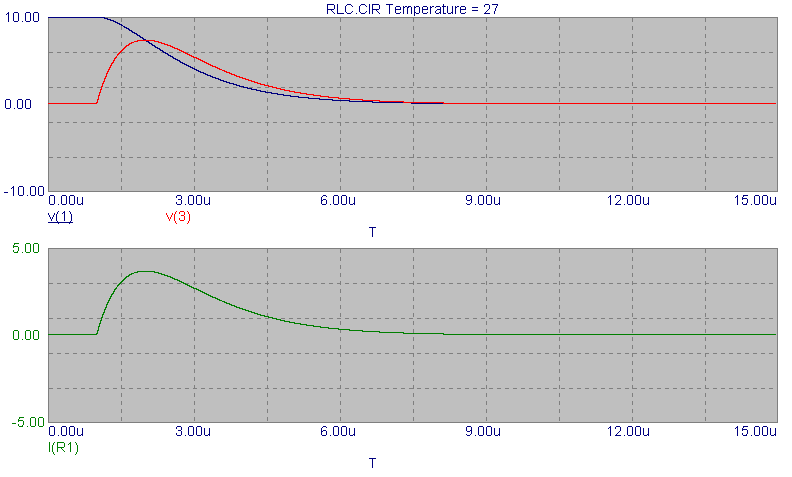Current V0 is the initial voltage on the capacitor (V)  L is the circuit inductance (H)  R is the circuit resistance (W)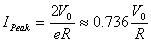Peak Current Same as above

##### Send consulting inquiries, comments, and suggestions to nessengr@san.rr.com

Ness Engineering, Inc.
P.O. Box 261501
San Diego, CA 92196
(858) 566-2372
(858) 240-2299 FAX

 © Richard M. Ness and Ness Engineering, Inc. Last Updated: